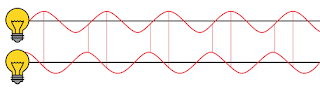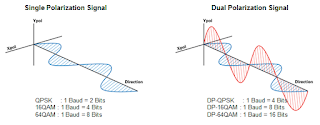## You need to test, we're here to help.

You need to test, we're here to help.

## 18 February 2016

### The Fundamentals of Coherent SignalsFigure 1: These two coherent light waves have a constant phase offset
Optical communications have come a long way from the simple direct detection of amplitude-modulated transmissions. Today's long-haul optical networks make use of coherent detection, and for good reason. Using coherent detection, receivers can track the phase of the incoming signal and thereby extract any information conveyed using phase and/or frequency modulation. Thus, coherent detection facilitates much higher capacity without using more bandwidth.

So just what is a "coherent signal?" By definition, a coherent signal is a pair of optical signals that have the same frequency and that maintain a constant phase offset. Coherent modulation formats modulate the phase of the coherent light wave (Figure 1). The phase is typically cyclic and relative, with phase information ranging from 0° to 360° or zero to 2Π. The phase-modulated information is typically recovered by comparing the received signal to a reference local oscillator in the form of an unmodulated light wave. Coherent signals can be modulated by either phase or by amplitude.Figure 2: Coherent modulation can be visualized in a constellation diagram or in separate I and Q eye diagrams
Coherent modulation is often visually represented using a constellation diagram of the in-phase and quadrature components of the signal (Q vs. I plot). This can also be thought of as the real and imaginary parts of the signal. In the constellation diagram, the angle of rotation on the circle represents the phase while distance from the center represents amplitude. Alternately, the symbol transitions can be visualized in terms of separate I (horizontal; real part) and Q (vertical; imaginary part) eye diagrams (Figure 2).

Quadrature phase-shift keying (QPSK) modulation is a favored format for coherent signals. QPSK modulation doubles the data rate of wavelength-division multiplexing (WDM) without increase in signal bandwidth by encoding two bits in each baud. Two bits enables four possible values for the signal, and the signal can be encoded at different phase components.Figure 3: When imposed on a QPSK signal, polarizationmultiplexing enables one baud to carry four bits
So if moving from WDM to QPSK doubles the data rate for a given bandwidth, let's double down again by imposing polarization multiplexing on our coherent signal. In addition to phase-encoding the signal, we can also polarize and split the electromagnetic wave into X and Y polarization planes, gaining a further multiplier of our bit rate. Thus, in QPSK, one baud conveys two bits, but with a dual polarization signal, one baud carries four bits (Figure 3).

Another modulation format known as quadrature amplitude modulation enables us to encode three different signal levels in a 16QAM signal, with one baud already carrying four bits. With dual polarization, a 16QAM signal contains eight bits in each baud. For 64QAM, eight bits would again double the dual polarization data rate to 16 bits/baud.

Thus, DP-QPSK quadruples the WDM data rate by encoding four bits in each baud with no increase in signal bandwidth. This modulation scheme, when added to the benefits of using coherent signal transmission over existing fiber, has yielded today's 40-Gbit/s and 100-Gbit/s coherent systems, with 200 Gbits/s and even 400 Gbits/s on the horizon for long-haul transmission. The 100-Gbit/s effective data rate is accomplished using 25-Gbaud DP-QSPK, which means a symbol rate of 25 Gbits/s, but our 4X multiplier brings us to the effective 100-Gbit/s data rate.

Our next installment will bring us to the means of analyzing these complex modulation formats: the optical modulation analyzer, or OMA.